• 举例求解矩阵的特征值和特征向量 （先明确：只有方阵才能出特征值，非方阵只能奇异值）   直接举一个例子：下面矩阵M的特征值和特征向量。 M=[460−3−50−3−61] M =\begin{bmatrix} 4 & 6 &...
举例求解矩阵的特征值和特征向量
（先明确：只有方阵才能求出特征值，非方阵只能求奇异值。）
直接举一个例子：求下面矩阵M的特征值和特征向量。
$M =\begin{bmatrix} 4 & 6 & 0 \\ -3 & -5 & 0 \\ -3 & -6 & 1 \end{bmatrix}$
设矩阵M的特征值为$\lambda$,列出矩阵M的特征方程：矩阵M减去，$\lambda$乘以和M同等大小的单位矩阵E。再让它们整体的行列式的值等于0即可。如下所示：
$|M - \lambda E| = 0\tag{1}$
求解(1)式的步骤如下：
$|M - \lambda E| = \begin{bmatrix} 4-\lambda & 6 & 0 \\ -3 & -5-\lambda & 0 \\ -3 & -6 & 1-\lambda \end{bmatrix} = (1-\lambda) \begin{bmatrix} 4-\lambda & 6 \\ -3 & -5-\lambda \end{bmatrix} = (\lambda -1)^{2}(\lambda + 2)$
所以特征值为1和-2，重数是1。
然后，把每个特征值$\lambda$带入到下方的线性方程组$(M - \lambda E)x = 0$，求出特征向量。
当$\lambda_{1}=-2$时，解线性方程组：$(M - (-2)E)x = 0$。
$|M + 2E| = \begin{bmatrix} 6 & 6 & 0 \\ -3 & -3 & 0 \\ -3 & -6 & 3 \end{bmatrix} \xRightarrow{先变成增广矩阵} \begin{bmatrix} 6 & 6 & 0 & 0\\ -3 & -3 & 0 & 0\\ -3 & -6 & 3 & 0 \end{bmatrix} \xRightarrow{再初等行变换} \begin{bmatrix} 1 & 1 & 0 & 0\\ 0 & 0 & 0 & 0\\ 0 & -1 & 1 & 0 \end{bmatrix}$
所以，$x_{1}+x_{2}=0; -x_{2}+x_{3}=0$。随便带一个值，一般取$x_{3}=1$，可解得$x_{2}=1，x_{1}=-1。$
解得：$x_{1}=-1，x_{2}=1，x_{3}=1$，所以$\lambda_{1}$的特征向量为：$p_{1} =\begin{bmatrix}-1\\ 1\\ 1\end{bmatrix}。$
当$\lambda_{2}=\lambda_{3}=1$时，解线性方程组：$(M - E)x = 0$。
$|M - E| = \begin{bmatrix} 3 & 6 & 0 \\ -3 & -6 & 0 \\ -3 & -6 & 0 \end{bmatrix} \xRightarrow{先变成增广矩阵} \begin{bmatrix} 3 & 6 & 0 & 0\\ -3 & -6 & 0 & 0\\ -3 & -6 & 0 & 0 \end{bmatrix} \xRightarrow{再初等行变换} \begin{bmatrix} 1 & 2 & 0 & 0\\ 0 & 0 & 0 & 0\\ 0 & 0 & 0 & 0 \end{bmatrix}$
所以，$x_{1}+2x_{2}=0; x_{3}=0$。随便带一个值，这里取$x_{1}=-2$，可解得$x_{2}=1。$
解得：$x_{1}=-2，x_{2}=1，x_{3}=0$，所以$\lambda_{2}$特征向量为：$p_{2} = \begin{bmatrix}-2\\ 1\\ 0\end{bmatrix}。$
再取，$x_{1}=x_{2}=0，x_{3}=1$，可解得$\lambda_{3}$的特征向量为：$p_{3} = \begin{bmatrix}0\\ 0\\ 1\end{bmatrix}。$
综上所述：矩阵M的特征值为$\lambda_{1}=-2，\lambda_{2}=\lambda_{3}=1$。对应的特征向量为：$p_{1} =\begin{bmatrix}-1\\ 1\\ 1\end{bmatrix}$、$p_{2} = \begin{bmatrix}-2\\ 1\\ 0\end{bmatrix}、p_{3} =\begin{bmatrix}0\\ 0\\ 1\end{bmatrix}$。
如何再继续进行矩阵的特征分解呢？
接下来进行矩阵的特征分解，上面已经求出了矩阵M的特征值和特征向量了，下面就非常简单了。
设矩阵Q为矩阵M的特征向量按列组成的矩阵。将特征向量按照从大到小、从左到右按列排列构成Q。 Λ 是对角矩阵，其对角线上的元素为对应的特征值。(按照刚才特征向量的位置，把对应特征值从上往下排列)如下所示：
$Q= \begin{bmatrix} -2 & 0 &-1\\ 1 & 0 &1 \\ 0 & 1 &1 \end{bmatrix} ，Λ= \begin{bmatrix} 1 & 0 &0\\ 0 & 1 &0 \\ 0 & 0 &-2 \end{bmatrix} 。$
所以最后，方阵M的特征值分解为：
$M=QΛQ^{-1}= \begin{bmatrix} -2 & 0 &-1\\ 1 & 0 &1 \\ 0 & 1 &1 \end{bmatrix} \begin{bmatrix} 1 & 0 &0\\ 0 & 1 &0 \\ 0 & 0 &-2 \end{bmatrix} \begin{bmatrix} -2 & 0 &-1\\ 1 & 0 &1 \\ 0 & 1 &1 \end{bmatrix}^{-1}$

最后注意:
能够进行特征分解的矩阵，要满足以下两个条件：
1.如果矩阵的所有特征根都不相等，那么绝对可以特征分解。
2.如果矩阵有等根,只需等根（重特征值）对应的那几个特征向量，是线性无关的。那么也可以特征分解。如果不是就不能了。
综合说：就是要有n个线性无关的特征向量。


展开全文线性代数 算法 机器学习 深度学习
• 即利用特征多项式可以出所有的特征值， 特征值之和等于原矩阵对角线元素之和 特征值的乘积等于原矩阵A的行列式的值。 特征多项式的乘积等于矩阵之积。 2、具体例子的求解方法 计算：A的特征值和特征向量。 ...
一、特征值特征向量定义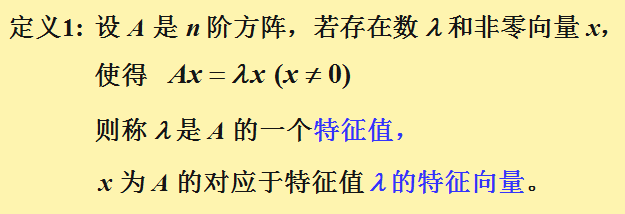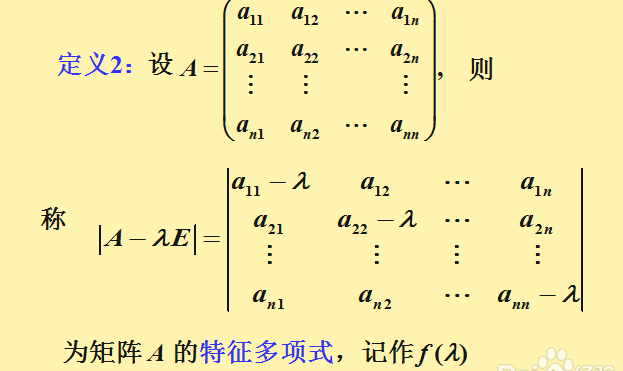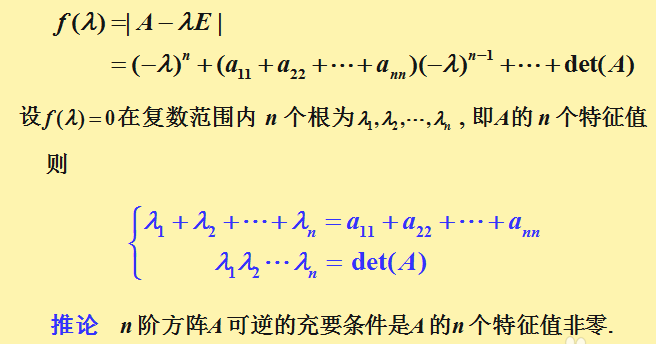即利用特征多项式可以求出所有的特征值，
特征值之和等于原矩阵对角线元素之和
特征值的乘积等于原矩阵A的行列式的值。
特征多项式的乘积等于矩阵之积。
2、具体例子的求解方法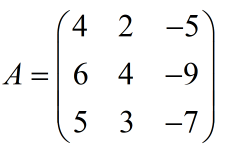计算：A的特征值和特征向量。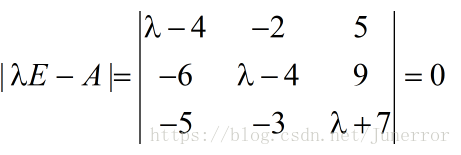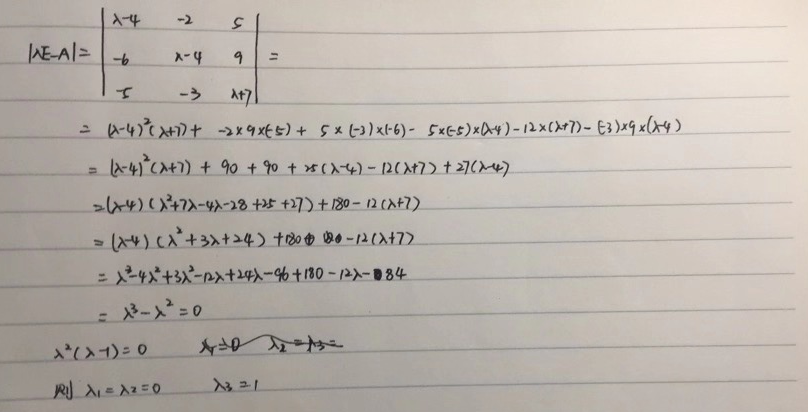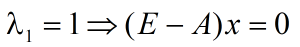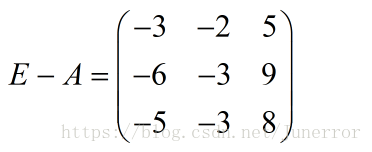化简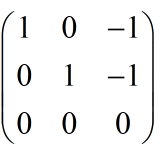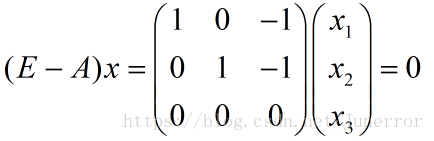令x=1，便可得出一个基础解系：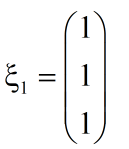同理当$λ_2=λ_3=0$时，得出：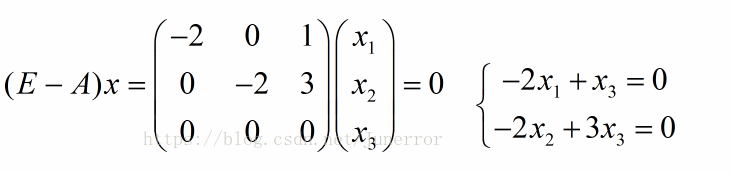同样可以得出特征向量：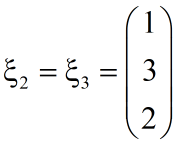展开全文• 学工科的小伙伴都学过线性代数，知道矩阵特征值、特征向量的定义及计算方法。不知道有没有小伙伴跟我一样对矩阵特征值和特征...我们个矩阵A的协方差阵Cov(A,A')，得到一个方阵B，而我们平时求的特征值，就是...
学工科的小伙伴都学过线性代数，知道求矩阵特征值、特征向量的定义及计算方法。不知道有没有小伙伴跟我一样对矩阵特征值和特征向量究竟是个什么东西而疑惑过，反正直到大学毕业之后的一两年内，我都没明白它到底有什么物理意义。在学了主元分析（PCA）后，暂且有所理解，这里跟大家分享一下，或有不对请指教。
事实上，我们求方阵特征值和特征向量是在处理一个半成品，对于一个非方阵的矩阵A，它往往代表一个多维空间里的多个数据。我们求这个矩阵A的协方差阵Cov(A,A’)，得到一个方阵B，而我们平时求的特征值，就是求B的特征值，那么求出的特征值是代表什么呢？它就是代表矩阵A的那些数据，在那个多维空间中，各个方向上分散的一个度量（即理解为它们在各个方向上的特征是否明显，特征值越大，则越分散，也就是特征越明显），而对应的特征向量，不言而喻，也就是它们对应的各个方向。
这是一个简单的对特征在物理意义上的理解，针对不同场合，它应当有更具体更实际的意义，希望对正在学习高等数学的小伙伴有所帮助。


展开全文线性代数
• matlab代码含参量矩阵特征值 普赛卡斯 Psecas（带自动求解器伪谱特征值计算器）是组使用伪谱方法求解特征值问题 (EVP) 方法。 Psecas 是为解决在天体物理流体动力学中执行线性稳定性计算时经常出现那种...
• 在学习使用PCA方法进行数据降维时，用到了特征值和特征向量知识。...上面用到了一个数学知识，在求矩阵A的特征值时，用到了： Ax=ax 其中，A是矩阵，a是特征值，x是特征向量。矩阵A的特征值可以有多个，特征值
在学习使用PCA方法进行数据降维时，用到了特征值和特征向量知识。
知乎链接，讲的太好了： https://www.zhihu.com/question/21874816

因为禁止转载，又想收藏，故采用截图方式保留作者思路。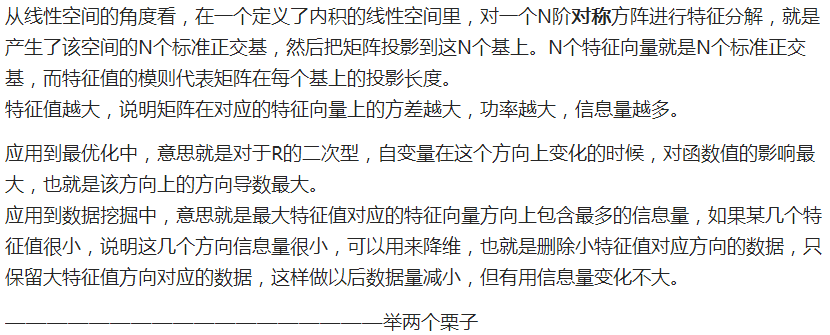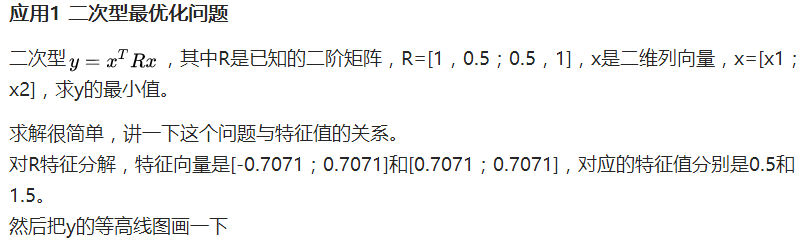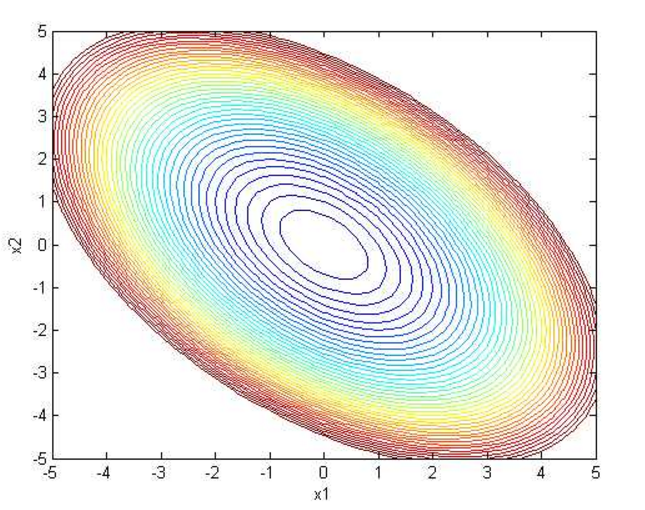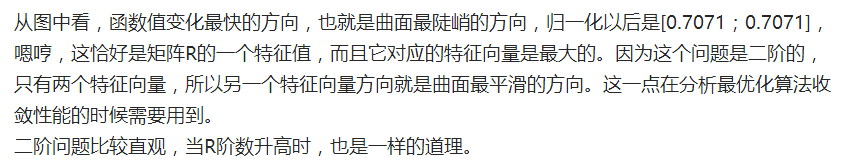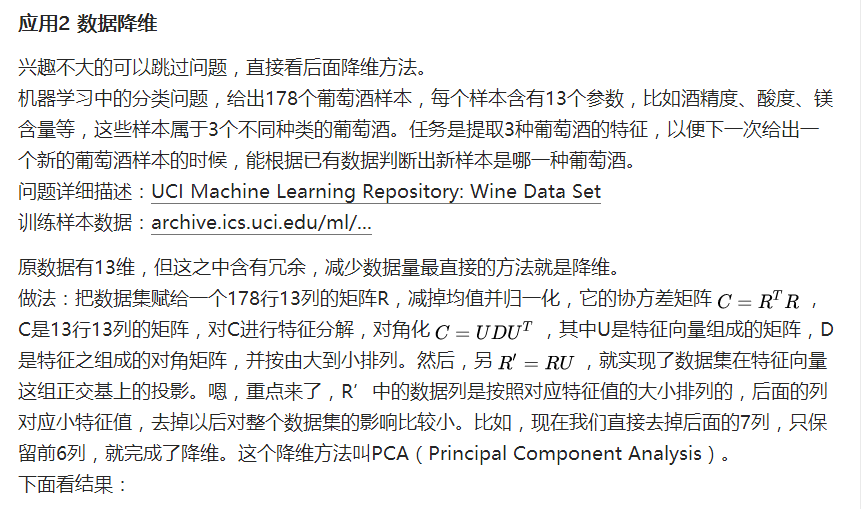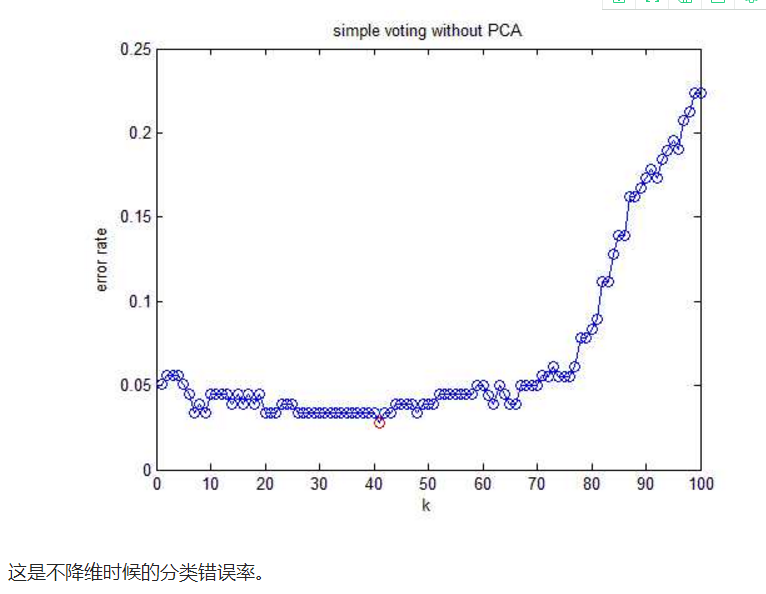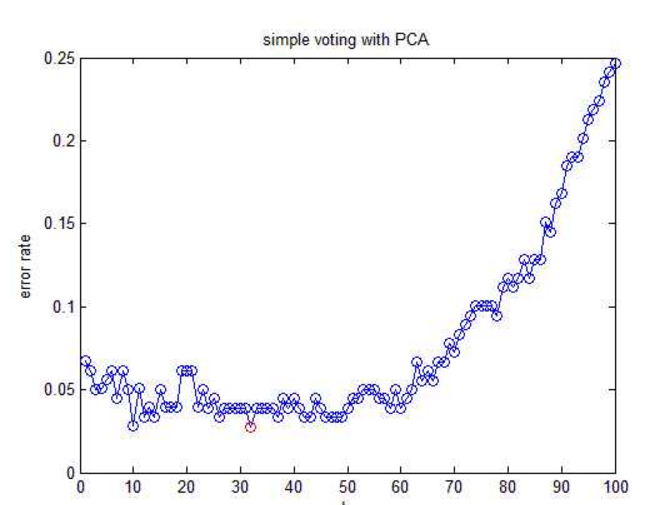上面用到了一个数学知识，在求矩阵A的特征值时，用到了：
Ax=ax
其中，A是矩阵，a是特征值，x是特征向量。矩阵A的特征值可以有多个，特征值越大，所对应的特征向量包含的信息量越大。
展开全文数学
• 关于矩阵的特征值，学习过线性代数的读者都知道一个公式AX=bX（b是所求的特征向量）现在我们假设A的矩阵是3阶方阵：[1 1/5 3; 5 1 6; 1/3 1/6 1]。下面我们看看matlab中的代码是如何求出这个判断矩阵的最大特征值。 ...
• 问题描述：给定一个矩阵，如下： A=[a11a21a12a22] A=\begin{bmatrix} ...那么如何求解此矩阵的特征值以及特征向量呢？这里我们要用到雅克比旋转。雅克比旋转Jacobi方法是对称矩阵的全部特征值以及相应的图像处理
• 特征值和特征向量意义 从定义出发，Ax=cx：A为n阶矩阵，c为特征值，x为特征向量。 矩阵A乘以x表示，对向量x进行一次转换（旋转或拉伸）（是一...这样做意义在于，看清一个矩阵在那些方面能产生最大效果（powe...
• 给定一个矩阵(没有任何特殊性质)，如何求的特征多项式？ 算法一 直接把$$\lambda$$代入$$(n+1)$$个点，求完行列式之后插值即可。 时间复杂度$$O(n^4)$$ 算法二 下面介绍一个更快的做法。 定义 对于矩阵\(\bm A,\...
• 特征向量和特征值在计算机视觉和机器学习中有...在这篇文章中，我将简单的介绍这个数学概念，并且展示如何手动获取二维方形矩阵的特征值分解。 特征向量是一个向量，当在它上面应用线性变换时其方向保持不变。考虑下面
• **问题：**如何求斐波那契数列通项？ notes：斐波那契数列：后面项是前面两项求和。 eg：1，1，2，3，5，8，13，21。。。 **解答：**我们将斐波那契数列问题写成矩阵形式，即： [xk+2xk+1]=∗[xk+1xk]...线性代数 算法 抽象代数 数学建模
• 通俗易懂理解协方差 协方差，可以通俗易懂理解为：两个变量在...公式简单翻译一下是:如果有x,Y两个变量,每个时刻"X与其均值之差"剩以Y与其均值之差"得到一个乘积,再对这每时刻乘积求和并出均值(其实是"期望
• 本文转自知乎大牛。 从定义出发，Ax=cx：A为矩阵，c为特征值，x为特征向量。 矩阵A乘以x表示，对向量x进行一次转换（旋转或拉伸）（是一...这样做意义在于，看清一个矩阵在那些方面能产生最大效果（power），并...
• 特征值和特征向量实际意义 从定义出发，Ax=cx：A为矩阵，c为特征值，x为特征向量。 矩阵A乘以x表示，对向量x进行一次转换（旋转或拉伸）（是一...这样做意义在于，看清一个矩阵在那些方面能产生最大效果（powe...
• 特征分解于奇异值分解的几何解释 在计算机图形学一中介绍了一些...特征分解其实就是在本科所学线代中一个矩阵的特征值与特征向量，然后利用二者进行一个相似对角化，这一节中考虑较为特殊的实对称矩阵，可以我...
• 从定义出发，Ax=cx：A为矩阵，c为特征值，x为特征向量。矩阵A乘以x表示，对向量x进行一次转换（旋转或拉伸）（是一种线性转换），而该转换...这样做意义在于，看清一个矩阵在那些方面能产生最大效果（power），...
• 称是线性变换的一个特征向量，是对应的特征值。 矩阵是一个表示二维空间的数组，矩阵可以看作是一个变换。在线性代数中，矩阵可以把一个向量变换到另一个位置，或者说从一个坐标系变换到另一个坐标系。矩阵的基，...
• RT，我在求一个超大矩阵（10021\*10021的稀疏图的邻接矩阵的特征值和特征向量遇到如下问题： (1)特征值和特征向量计算时间过长，虽然可以运行出来，但是需要处理大约50分钟，这里使用matlab自带的eig函数运算，...matlab 特征向量
• 一个向量x，乘上矩阵A后 Ax 中仍然平行于 x那部分，就是特征向量 特征值 这个λ\lambdaλ 就是特征值 例子：投影矩阵、置换矩阵 fact sum of the 特征值 is sum down the diagnal of A 如何求特征值 由于这个 ...线性代数
• 如何判断一个矩阵是否有非零特征值？ 设 $A$ 是 $n$ 阶方阵，则有 $A$ 有非零特征值 $\iff$ $A \ne 0$ 。 证明： $A$ 没有非零特征值 $\iff$ \$|\lambda I - A| = \lambda^n \iff (\lambda I - A) \sim \lambda I \...
• 2018-05-12请问如何用雅克比法求解矩阵特征值和特征向量雅可比方法的基本思想是通过系列的由平面旋转矩阵构成的正交变换将实对称矩阵逐步化为对角阵,从而得到 的全部特征值及其相应的特征向量。首先引进 中的平面...
• 文章目录方阵的行列式性质例题伴随矩阵(只有方阵才有伴随矩阵)定理逆矩阵的定义1)如何判断一个矩阵可逆？2)A−1=?A^{-1}=?A−1=?定理矩阵的方法例题逆矩阵的性质伴随矩阵A∗A^*A∗小结参考 线性代数请记住：......